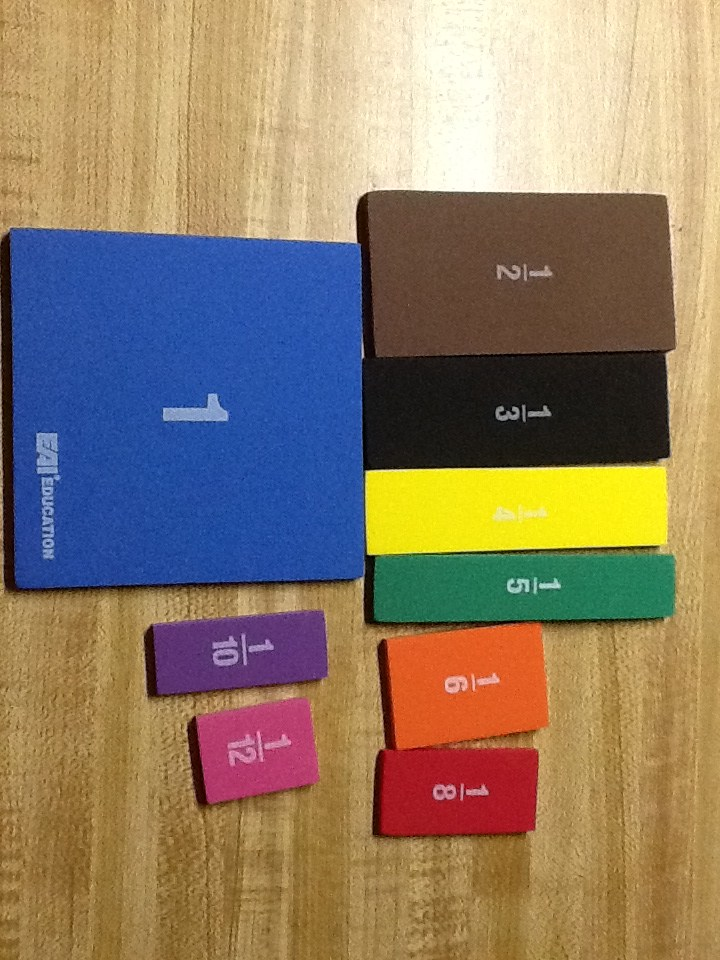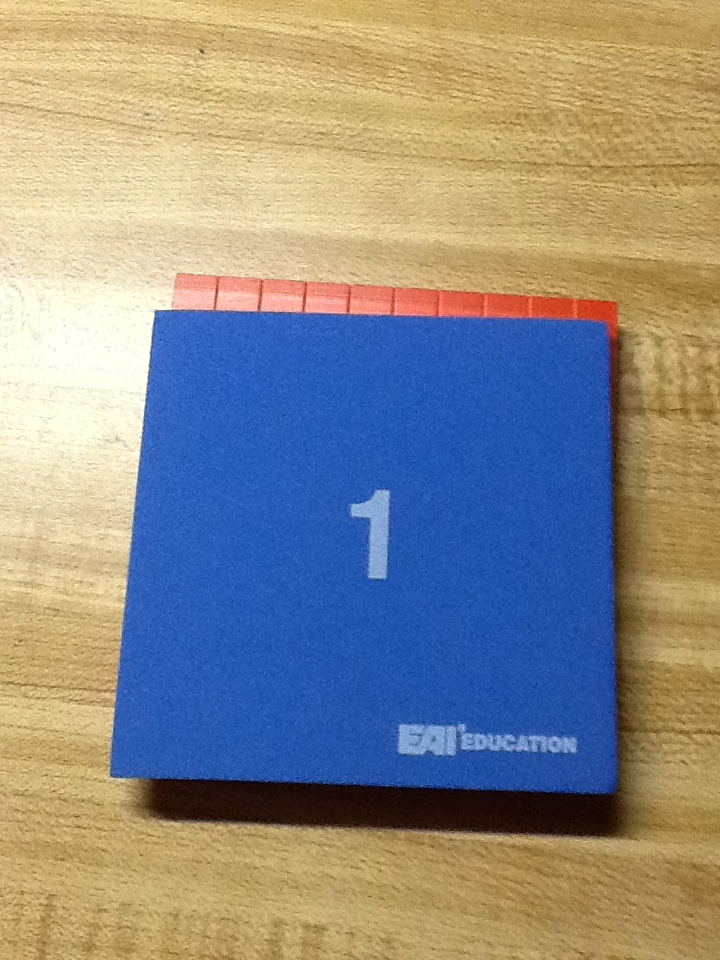# My Favorite Fraction Tools

I wanted to share with you these new tools that I ordered for this year.  I knew that we would be studying a lot of fractions, and we only had the linear models for a class set.  Normally, when introducing fractions I start out with whole sheets of colored paper and have students cut them apart into halves, thirds, fourths, and so on.  Then students can lay these pieces of paper on each other to find equivalent fractions.  While this has value for students understanding that fractions are parts of a whole (piece of paper), students tend not to cut them out perfectly, so their equivalence investigation is a bit skewed.  Because of this I move to manipulatives for the equivalence investigation, but again, I only had tools that were linear models…so here is what I ordered…  Foamy fraction squares!What is even better than the fact that they are brightly colored and quiet?They are exactly the same size as a base ten block!  This makes teaching decimal equivalents so much easier!  I know the shadow makes the base ten block look larger but, they really are the same size.

See!Students can easily see that one-half equals five-tenths, one-fifth equals two tenths, and one tenth equals ten hundredths.I ordered a class set of these from EAI education here.  I promise I make no money from telling you about these, but they make teaching fractions so much easier.  Every teacher who has used them loves them!